# Mason's gain formula for determining overall gain of system (with example)

## Mason's gain formula for determining overall gain of system

### Introduction:

In the last article we have seen block diagram algebra. For studying the complete behavior of the system we should know its transfer function. Using block diagram algebra we can reduce the block diagram of a control system into a single block representing the transfer function of that system. But in case of complicated block diagrams it will become difficult to reduce the block diagram into its equivalent single block having same input and output as that of given control system.

So to avoid this complexity of reducing the block diagram we use one simple method. In this method we first construct a signal flow graph of the given system using its ‘describing equations’. And using this signal flow graph and one formula (known as Mason’s gain formula) we can easily find out the overall gain of the given control system.

### Construction of signal flow graph:

As discussed earlier, for the construction of signal flow graph we need its set of describing equations. For the clear understanding of construction of signal flow graph, we are using following set of equations: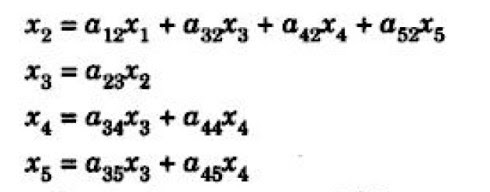Where x1 is the input variable and x5 is the output variable.

### Steps to draw signal flow graph:

1)      In the given set of instruction there are five nodes (x1, x2… x5), therefore mark five nodes as shown in diagram a) as below.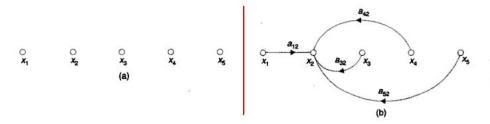2)      Now consider the first equation, in this equation x2 is equal to the sum of four signals having different coefficients.  When we connect x1 with x2 the direction should be indicated as (1?2) because of a12, similarly for x2 and x3 the arrow should be in the direction of (3?2) because of a32. The signal flow graph for the first equation is shown in the diagram b).

3)      Similarly, we have to follow the same procedure for all remaining equations, which are shown in figure c), d) and e).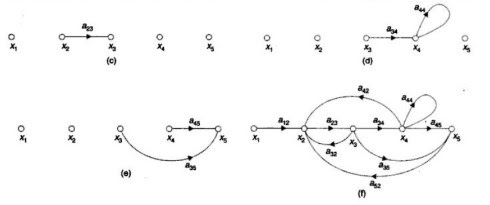4)      Finally figure f) shows the complete representation of the signal flow graph of the given control system.

### Mason’s gain formula:

As we know the relationship between the input and output of the given control system is given by its net gain. This net gain is also known as overall gain of the system. For determining the overall gain of the control system Mason’s derived one formula, this formula is used for calculating the overall gain of the system, which is given by,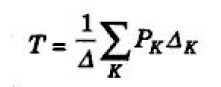Where,

• Pk represents path gain of kth forward path.

• ? represents determinant of the graph which is given by:

? = 1- (sum of loop gains of all individual loops) + (sum of gain products of all possible combinations of two non-touching loops) – (sum of gain products of all possible combinations of three non-touching loops) + …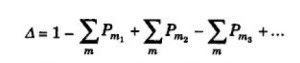• ‘?k’ represents the value of ? for that part of the graph which does not touch the kth forward path.

• ‘T’ is the overall gain of the given control system.

### Solution:

In the given signal flow graph x1 is the input variable, whereas x5 is the output variable.

By using Mason’s gain formula, overall gain of the system is given by,

1)      In the first step, we have to determine the number of forward path. Forward path means the path from input node to the output node. So in this case we have 2 forward path (i.e. k=2) with path gains: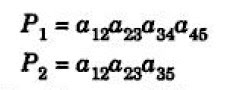2)      There are 5 individual loops with loop gains: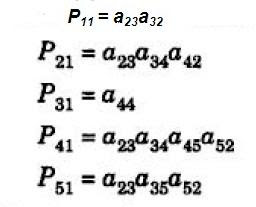3)      Now we have to find the all possible combinations of two non-touching loops (i.e. those loops which do not contain common node) and their gain products. So such non-touching loops with there product gain is given by: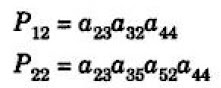4)      Similarly, we have to find all possible combination of three non-touching loops. But in this case there are no such combination exist.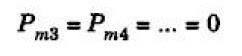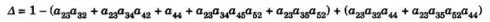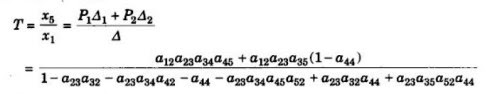### You may also like:

1.